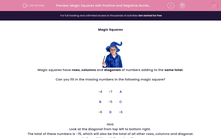# Understand Magic Squares with Positive and Negative Numbers

In this worksheet, students will solve problems with positive and negative numbers using 'Magic Squares'.This content is premium and exclusive to EdPlace subscribers.Key stage:  KS 2

Curriculum topic:   Maths and Numerical Reasoning

Curriculum subtopic:   Mixed Problems

Difficulty level:#### Worksheet Overview

Magic SquaresMagic squares have rows, columns and diagonals of numbers adding to the same total.

Can you fill in the missing numbers in the following magic square?

 −4 −7 A B −5 C −6 D −6

Hint:

Look at the diagonal from top left to bottom right.

The total of these numbers is -15, which will also be the total of all other rows, columns and diagonal.

Let's work through these together:

A = −4

-7 + -4 = -11

-11 + -4 = -15

= −3

-6 + -6 = -12

-15 - -12 = -3

= −5

We have already worked out that A = -4.

-6 + -4 = -10

-15 - -10 = -5

B = −5

We have already worked out that C = -5.

-5 + -5 = -10

-15 - -15 = -5

Now, it's over to you!

### What is EdPlace?

We're your National Curriculum aligned online education content provider helping each child succeed in English, maths and science from year 1 to GCSE. With an EdPlace account you’ll be able to track and measure progress, helping each child achieve their best. We build confidence and attainment by personalising each child’s learning at a level that suits them.

Get started